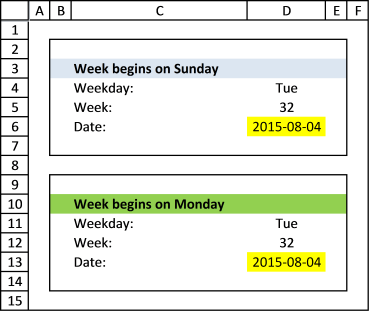Author: Oscar Cronquist Article last updated on February 02, 2018

I want to find day if given date and week, for example : if given Tuesday, 32th week, 2015 >>> how to use function to point out 4/8/2015 ?Formula in cell D6:

=DATE(YEAR(TODAY()), 1, 1)-WEEKDAY(DATE(YEAR(TODAY()), 1, 1), 1)+(7*(D5-1))+MATCH(\$D\$4, {"Sun", "Mon", "Tue", "Wed", "Thu", "Fri", "Sat"}, 0)

Formula in cell D13:

=DATE(YEAR(TODAY()), 1, 1)-WEEKDAY(DATE(YEAR(TODAY()), 1, 1), 2)+(7*(D12-1))+MATCH(\$D\$11, {"Mon", "Tue", "Wed", "Thu", "Fri", "Sat", "Sun"}, 0)

### Explaining formula in cell D6

Step 1 - Calculate the first date of this year

DATE(YEAR(TODAY()), 1, 1) returns 42005

Step 2 - Subtract with the corresponding weekday number

The weekday function converts a date to a number ranging from 1 to 7, depending on when the week starts.

WEEKDAY(serialnumber, return_type)

If you live in the US the week in starts on Sundays, return_type is 1.

-WEEKDAY(DATE(YEAR(TODAY()), 1, 1), 1)

becomes WEEKDAY(42005, 1)

and returns 5. 42005 is 2015-01-01 and that day is a thursday.

Step 3 - Calculate and add the number of days from monday/week 1 to monday/(nth week -1)

+(7*(D5-1))

Example, 7*(32-1)

becomes

7*31 and returns 217.

Step 4 - Calculate and add weekday number

MATCH(\$D\$4, {"Sun", "Mon", "Tue", "Wed", "Thu", "Fri", "Sat"}, 0)

If you week begins on sunday, this is the array you want to be using:

{"Sun", "Mon", "Tue", "Wed", "Thu", "Fri", "Sat"}.

Example, Tue returns 3.

Step 5 - Add and subtract

42005-5+217+3 = 42220

42220 is 2015-08-04